Question

A hockey puck of mass 0.39 kg is shot west at 2.90 m/s strikes a second puck, initially at rest, of mass 0.55 kg. As a result of the collision, the first puck is deflected at an angle of 33° south of west, with a speed of 1.45 m/s. What is the speed of the second puck after the collision?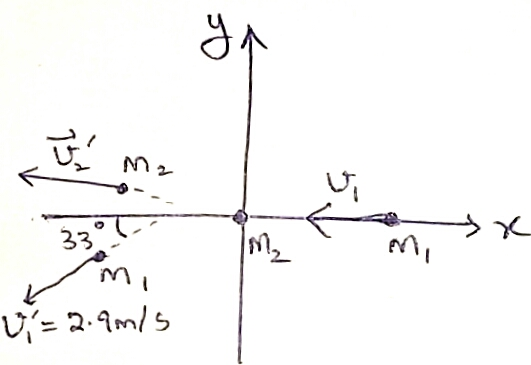Initial momentum of the first puck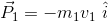Initially the second particle is at rest, It's initial momentum is zero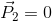Total initial momentum of the system is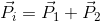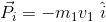The final momentum of the second particle is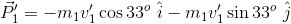Let v​​​​​​2​​​' be the final velocity of the second particle. The final momentum of the second particle is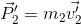Total final momentum of the system is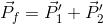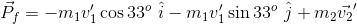By the conservation of momentum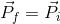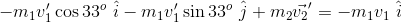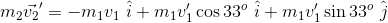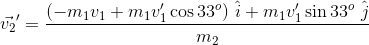Substituting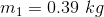,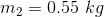,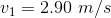,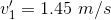we get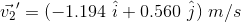The speed of the second particle after the collision is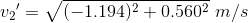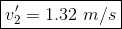#### Earn Coins

Coins can be redeemed for fabulous gifts.

Similar Homework Help Questions
• ### A hockey puck (1) of mass 170 g is shot west at a speed of 6.60...

A hockey puck (1) of mass 170 g is shot west at a speed of 6.60 m/s. It strikes a second puck (2), initially at rest, of mass 195 g. As a result of the collision, the first puck (1) is deflected at an angle of 62° north of west and the second puck (2) moves at an angle of 50° south of west. What is the magnitude of the velocity of puck (1) after the collision?

• ### 8. A hockey puck (1) of mass 130 g is shot west at a speed of...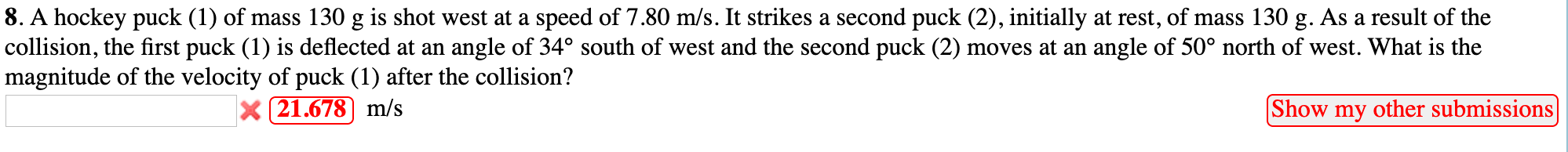8. A hockey puck (1) of mass 130 g is shot west at a speed of 7.80 m/s. It strikes a second puck (2), initially at rest, of mass 130 g. As a result of the collision, the first puck (1) is deflected at an angle of 34° south of west and the second puck (2) moves at an angle of 50° north of west. What is the magnitude of the velocity of puck (1) after the collision? x 21.678...

• ### A hockey puck (1) of mass 180 g is shot east at a speed of 8.80...

A hockey puck (1) of mass 180 g is shot east at a speed of 8.80 m/s. It strikes a second puck (2), initially at rest, of mass 198 g. As a result of the collision, the first puck (1) is deflected at an angle of 30° south of east and the second puck (2) moves at an angle of 50° north of east. What is the magnitude of the velocity of puck (1) after the collision? m/s

• ### A hockey puck (1) of mass 120 g is shot east at a speed of 8.80...

A hockey puck (1) of mass 120 g is shot east at a speed of 8.80 m/s. It strikes a second puck (2), initially at rest, of mass 138 g. As a result of the collision, the first puck (1) is deflected at an angle of 46° south of east and the second puck (2) moves at an angle of 40° north of east. What is the magnitude of the velocity of puck (1) after the collision?

• ### 6. An explosion breaks an object initially at rest into two pieces, one of which has...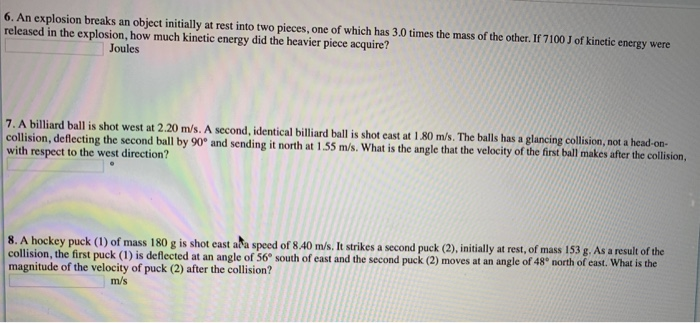6. An explosion breaks an object initially at rest into two pieces, one of which has 3.0 times the mass of the other. If 7100 J of kinetic energy were released in the explosion, how much kinetic energy did the heavier piece acquire? Joules 7. A billiard ball is shot west at 2.20 m/s. A second, identical billiard ball is shot cast at 1.80 m/s. The balls has a glancing collision, not a head-on- collision, deflecting the second ball by...

• ### A hockey puck of mass 0.16 kg, sliding on a nearly frictionless surface of ice with...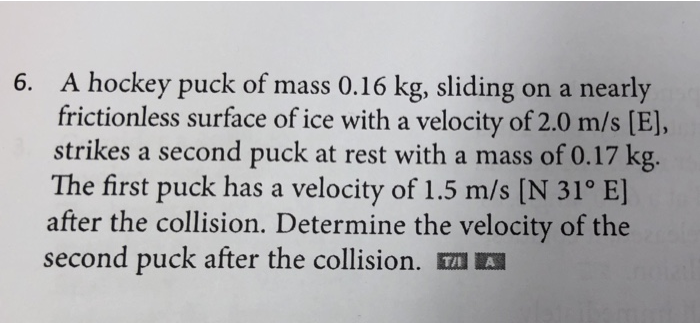A hockey puck of mass 0.16 kg, sliding on a nearly frictionless surface of ice with a velocity of 2.0 m/s [E], strikes a second puck at rest with a mass of 0.17 kg. The first puck has a velocity of 1.5 m/s [N 31o E] after the collision. Determine the velocity of the second puck after the collision. TA 6.

• ### A hockey puck of mass m traveling along the x axis at 4.5 m/s hits another...

A hockey puck of mass m traveling along the x axis at 4.5 m/s hits another identical hockey puck at rest. If after the collision the second puck travels at a speed of 3.5 m/s at an angle of 30° above the x axis, what is the final velocity of the first puck?

• ### Hockey puck impacts another puck.

A hockey puck with a mass 0.250 kg traveling along a line at 1.5 m/s strikes a stationary puck with the same mass. The first puck exits the collision in a directionthat is 30 degrees away from the line at a speed of 0.75 m/s. What is the direction and magnitude of the velocity of the second puck after the collision? Is this anelastic collision? Include all formulas used and show work clearly. Thanks!

• ### Hockey puck A is shot along the x-axis at 5.60 m/s at identicalhockey puck B...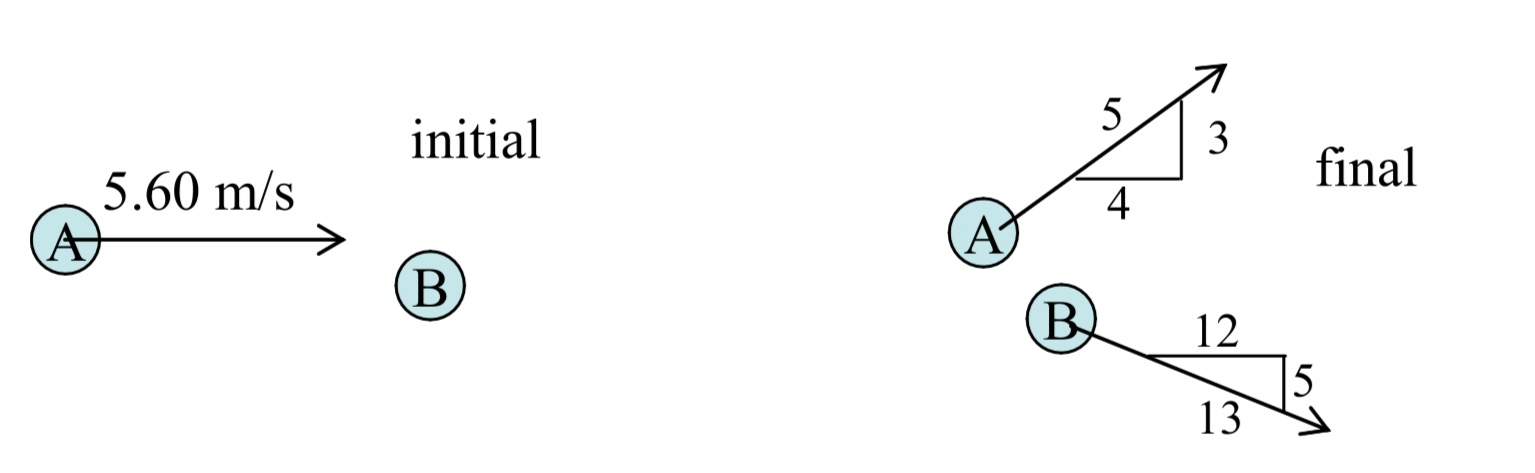Hockey puck A is shot along the x-axis at 5.60 m/s at identical hockey puck B which is initially at rest on horizontal frictionless ice. The collision is off-center, and they scatter at the angles shown.a)  Find the speeds vA2 and vB2 of the pucks after the collision.b)  Find the percentage of kinetic energy converted into other forms during this collision.c)  Determine the coefficient of restitution for this collision. (Note: Since B is at rest initially, the...

• ### 20. A hockey puck travels across the ice at a speed of 38 m/s 34° north...

20. A hockey puck travels across the ice at a speed of 38 m/s 34° north of east. It has a glancing collision with a stationary puck of the exact same mass. The previously stationary puck moves at 33 m/s 24° north of east. What is the speed and direction of the first puck (both pucks have a mass of 0.3 kg) after the collision? Is the collision elastic or inelastic?